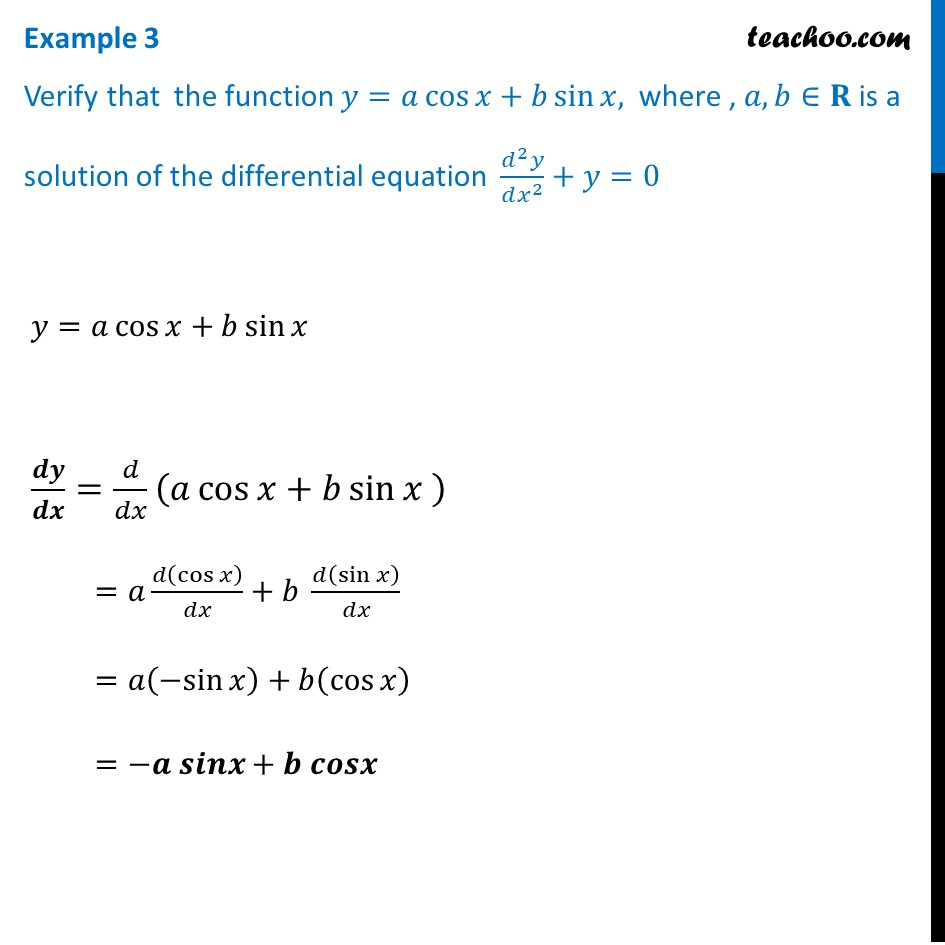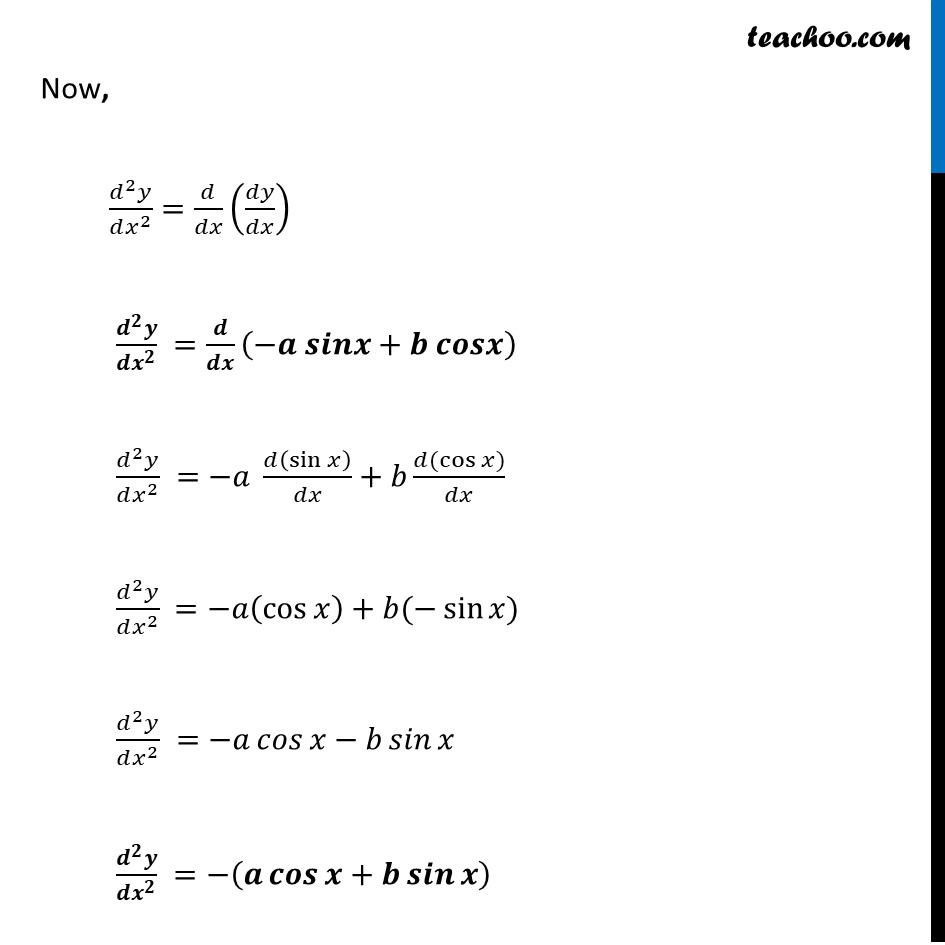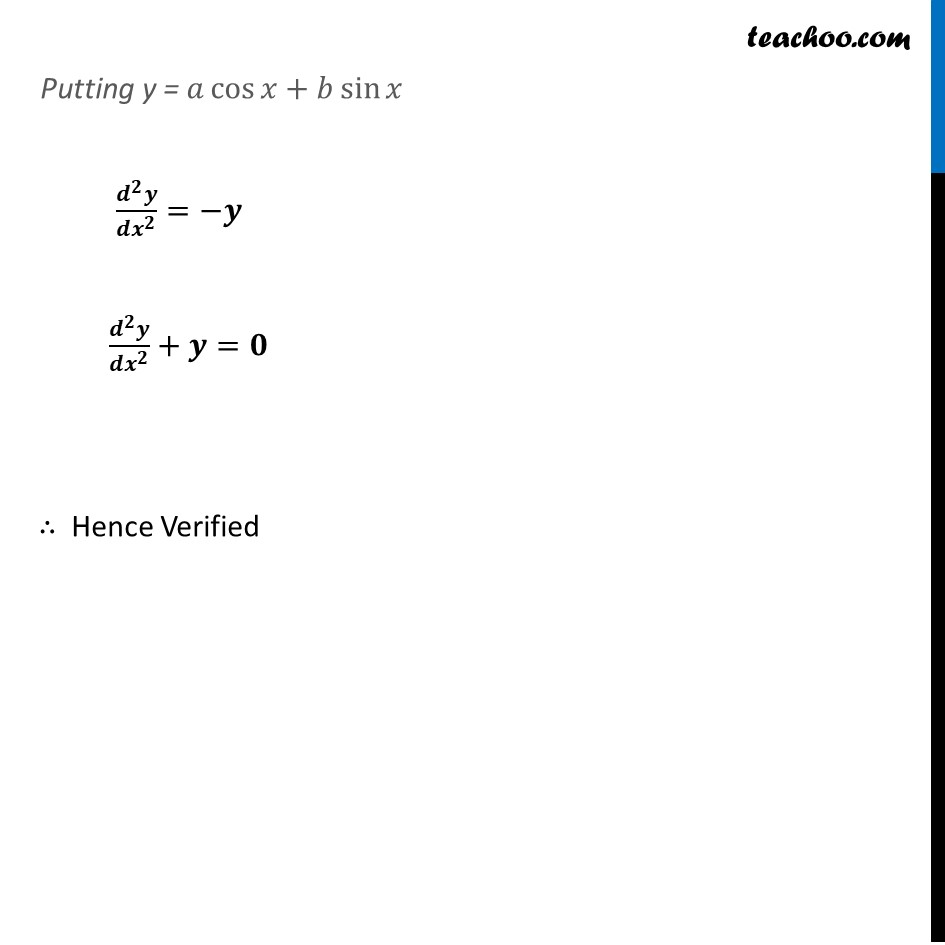Examples

Chapter 9 Class 12 Differential Equations
Serial order wiseLearn in your speed, with individual attention - Teachoo Maths 1-on-1 Class

### Transcript

Example 3 Verify that the function 𝑦=𝑎 cos⁡〖𝑥+𝑏 sin⁡〖𝑥, 〗 〗 where , 𝑎, 𝑏∈𝐑 is a solution of the differential equation (𝑑^2 𝑦)/(𝑑𝑥^2 )+𝑦=0 𝑦=𝑎 cos⁡〖𝑥+𝑏 sin⁡〖𝑥 〗 〗 𝒅𝒚/𝒅𝒙=𝑑/𝑑𝑥 (𝑎 cos⁡〖𝑥+𝑏 sin⁡〖𝑥 〗 〗 ) =𝑎 𝑑(cos⁡𝑥 )/𝑑𝑥+𝑏 𝑑(sin⁡𝑥 )/𝑑𝑥 =𝑎(〖−sin〗⁡𝑥 )+𝑏(cos⁡𝑥 ) =−𝒂 𝒔𝒊𝒏𝒙+𝒃 𝒄𝒐𝒔𝒙 Now, (𝑑^2 𝑦)/(𝑑𝑥^2 )=𝑑/𝑑𝑥 (𝑑𝑦/𝑑𝑥) (𝒅^𝟐 𝒚)/(𝒅𝒙^𝟐 ) =𝒅/𝒅𝒙 (−𝒂 𝒔𝒊𝒏𝒙+𝒃 𝒄𝒐𝒔𝒙) (𝑑^2 𝑦)/(𝑑𝑥^2 ) =−𝑎 𝑑(sin⁡𝑥 )/𝑑𝑥+𝑏 (𝑑(cos⁡𝑥))/𝑑𝑥 (𝑑^2 𝑦)/(𝑑𝑥^2 ) =−𝑎(cos⁡𝑥 )+𝑏(−sin⁡𝑥) (𝑑^2 𝑦)/(𝑑𝑥^2 ) =−𝑎 𝑐𝑜𝑠⁡〖𝑥−𝑏 𝑠𝑖𝑛⁡𝑥 〗 (𝒅^𝟐 𝒚)/(𝒅𝒙^𝟐 ) =−(𝒂 𝒄𝒐𝒔⁡〖𝒙+𝒃 𝒔𝒊𝒏⁡𝒙 〗 ) Putting y = 𝑎 cos⁡〖𝑥+𝑏 sin⁡〖𝑥 〗 〗 (𝒅^𝟐 𝒚)/(𝒅𝒙^𝟐 )=−𝒚 (𝒅^𝟐 𝒚)/(𝒅𝒙^𝟐 )+𝒚=𝟎 ∴ Hence Verified